# rocmetrics

Receiver operating characteristic (ROC) curve and performance metrics for binary and multiclass classifiers

Since R2022a

## Description

Create a `rocmetrics` object to evaluate the performance of a classification model using receiver operating characteristic (ROC) curves or other performance metrics. `rocmetrics` supports both binary and multiclass problems.

For each class, `rocmetrics` computes performance metrics for a one-versus-all ROC curve. You can compute metrics for an average ROC curve by using the `average` function. After computing metrics for ROC curves, you can plot them by using the `plot` function.

By default, `rocmetrics` computes the false positive rates (FPR) and the true positive rates (TPR) to obtain a ROC curve and the area under the ROC curve (AUC). You can compute additional metrics by specifying the `AdditionalMetrics` name-value argument when you create an object or by calling the `addMetrics` function after you create an object. A `rocmetrics` object stores the computed metrics and AUC values in the `Metrics` and `AUC` properties, respectively.

`rocmetrics` computes pointwise confidence intervals for the performance metrics when you set the `NumBootstraps` value to a positive integer or when you specify cross-validated data for the true class labels (`Labels`), classification scores (`Scores`), and observation weights (`Weights`). For details, see Pointwise Confidence Intervals.

## Creation

### Syntax

``rocObj = rocmetrics(Labels,Scores,ClassNames)``
``rocObj = rocmetrics(Labels,Scores,ClassNames,Name=Value)``

### Description

example

````rocObj = rocmetrics(Labels,Scores,ClassNames)` creates a `rocmetrics` object using the true class labels in `Labels` and the classification scores in `Scores`. Specify `Labels` as a vector of length n, and specify `Scores` as a matrix of size `n`-by-`K`, where `n` is the number of observations, and `K` is the number of classes. `ClassNames` specifies the column order in `Scores`.The `Metrics` and `AUC` properties contain the performance metrics and AUC value for each class for which you specify `Scores` and `ClassNames`.If you specify cross-validated data in `Labels` and `Scores` as cell arrays, then `rocmetrics` computes confidence intervals for the performance metrics. ```

example

````rocObj = rocmetrics(Labels,Scores,ClassNames,Name=Value)` specifies additional options using one or more name-value arguments. For example, `NumBootstraps=100` draws 100 bootstrap samples to compute confidence intervals for the performance metrics.```

### Input Arguments

expand all

True class labels, specified as a numeric vector, logical vector, categorical vector, character array, string array, or cell array of character vectors. You can also specify `Labels` as a cell array of one of these types for cross-validated data.

• For data that is not cross-validated, the length of `Labels` and the number of rows in `Scores` must be equal.

• For cross-validated data, you must specify `Labels`, `Scores`, and `Weights` as cell arrays with the same number of elements. `rocmetrics` treats an element in the cell arrays as data from one cross-validation fold and computes pointwise confidence intervals for the performance metrics. The length of `Labels{i}` and the number of rows in `Scores{i}` must be equal.

Each row of `Labels` or `Labels{i}` represents the true label of one observation.

This argument sets the `Labels` property.

Data Types: `single` | `double` | `logical` | `char` | `string` | `cell`

Classification scores, specified as a numeric matrix or a cell array of numeric matrices.

Each row of the matrix in `Scores` contains the classification scores of one observation for all classes specified in `ClassNames`. The column order of `Scores` must match the class order in `ClassNames`.

• For a matrix input, `Score(j,k)` is the classification score of observation `j` for class `ClassNames(k)`. You can specify `Scores` by using the second output argument of the `predict` function of a classification model object for both binary classification and multiclass classification. For example, `predict` of `ClassificationTree` returns classification scores as an n-by-K matrix, where n is the number of observations and K is the number classes. Pass the output to `rocmetrics`.

The number of rows in `Scores` and the length of `Labels` must be equal. `rocmetrics` adjusts scores for each class relative to the scores for the rest of the classes. For details, see Adjusted Scores for Multiclass Classification Problem.

• For a vector input, `Score(j)` is the classification score of observation `j` for the class specified in `ClassNames`.

• `ClassNames` must contain only one class.

• `Prior` must be a two-element vector with `Prior(1)` representing the prior probability for the specified class.

• `Cost` must be a `2`-by-`2` matrix containing `[Cost(P|P),Cost(N|P);Cost(P|N),Cost(N|N)]`, where `P` is a positive class (the class for which you specify classification scores), and `N` is a negative class.

• The length of `Scores` and the length of `Labels` must be equal.

If you want to display the model operating point when you plot the ROC curve using the `plot` function, the values in `Score(j)` must be the posterior probability. This restriction applies only to a vector input.

• For cross-validated data, you must specify `Labels`, `Scores`, and `Weights` as cell arrays with the same number of elements. `rocmetrics` treats an element in the cell arrays as data from one cross-validation fold and computes pointwise confidence intervals for the performance metrics. `Score{i}(j,k)` is the classification score of observation `j` in element `i` for class `ClassNames(k)`. The number of rows in `Scores{i}` and the length of `Labels{i}` must be equal.

This argument sets the `Scores` property.

Data Types: `single` | `double` | `cell`

Class names, specified as a numeric vector, logical vector, categorical vector, character array, string array, or cell array of character vectors. `ClassNames` must have the same data type as the true labels in `Labels`. The values in `ClassNames` must appear in `Labels`.

This argument sets the `ClassNames` property.

Data Types: `single` | `double` | `logical` | `cell` | `categorical`

Name-Value Arguments

Specify optional pairs of arguments as `Name1=Value1,...,NameN=ValueN`, where `Name` is the argument name and `Value` is the corresponding value. Name-value arguments must appear after other arguments, but the order of the pairs does not matter.

Example: `FixedMetric="FalsePositiveRate",FixedMetricValues=0:0.01:1` holds the FPR values fixed at `0:0.01:1`.

Performance Metrics

expand all

Additional model performance metrics to compute, specified as a character vector or string scalar of the built-in metric name, string array of names, function handle (`@metricName`), or cell array of names or function handles. A `rocmetrics` object always computes the false positive rates (FPR) and the true positive rates (TPR) to obtain a ROC curve. Therefore, you do not have to specify to compute FPR and TPR.

• Built-in metrics — Specify one of the following built-in metric names by using a character vector or string scalar. You can specify more than one by using a string array.

NameDescription
`"TruePositives"` or `"tp"`Number of true positives (TP)
`"FalseNegatives"` or `"fn"`Number of false negatives (FN)
`"FalsePositives"` or `"fp"`Number of false positives (FP)
`"TrueNegatives"` or `"tn"`Number of true negatives (TN)
`"SumOfTrueAndFalsePositives"` or `"tp+fp"`Sum of TP and FP
`"RateOfPositivePredictions"` or `"rpp"`Rate of positive predictions (RPP), `(TP+FP)/(TP+FN+FP+TN)`
`"RateOfNegativePredictions"` or `"rnp"`Rate of negative predictions (RNP), `(TN+FN)/(TP+FN+FP+TN)`
`"Accuracy"` or `"accu"`Accuracy, `(TP+TN)/(TP+FN+FP+TN)`
`"FalseNegativeRate"`, `"fnr"`, or `"miss"`False negative rate (FNR), or miss rate, `FN/(TP+FN)`
`"TrueNegativeRate"`, `"tnr"`, or `"spec"`True negative rate (TNR), or specificity, `TN/(TN+FP)`
`"PositivePredictiveValue"`, `"ppv"`, or `"prec"`Positive predictive value (PPV), or precision, `TP/(TP+FP)`
`"NegativePredictiveValue"` or `"npv"`Negative predictive value (NPV), `TN/(TN+FN)`
`"ExpectedCost"` or `"ecost"`

Expected cost, `(TP*cost(P|P)+FN*cost(N|P)+FP*cost(P|N)+TN*cost(N|N))/(TP+FN+FP+TN)`, where `cost` is a 2-by-2 misclassification cost matrix containing `[0,cost(N|P);cost(P|N),0]`. `cost(N|P)` is the cost of misclassifying a positive class (`P`) as a negative class (`N`), and `cost(P|N)` is the cost of misclassifying a negative class as a positive class.

The software converts the `K`-by-`K` matrix specified by the `Cost` name-value argument of `rocmetrics` to a 2-by-2 matrix for each one-versus-all binary problem. For details, see Misclassification Cost Matrix.

The software computes the scale vector using the prior class probabilities (`Prior`) and the number of classes in `Labels`, and then scales the performance metrics according to this scale vector. For details, see Performance Metrics.

• Custom metric — Specify a custom metric by using a function handle. A custom function that returns a performance metric must have this form:

`metric = customMetric(C,scale,cost)`

• The output argument `metric` is a scalar value.

• A custom metric is a function of the confusion matrix (`C`), scale vector (`scale`), and cost matrix (`cost`). The software finds these input values for each one-versus-all binary problem. For details, see Performance Metrics.

• `C` is a `2`-by-`2` confusion matrix consisting of `[TP,FN;FP,TN]`.

• `scale` is a `2`-by-`1` scale vector.

• `cost` is a `2`-by-`2` misclassification cost matrix.

The software does not support cross-validation for a custom metric. Instead, you can specify to use bootstrap when you create a `rocmetrics` object.

Note that the positive predictive value (PPV) is `NaN` for the reject-all threshold for which `TP` = `FP` = `0`, and the negative predictive value (NPV) is `NaN` for the accept-all threshold for which `TN` = `FN` = `0`. For more details, see Thresholds, Fixed Metric, and Fixed Metric Values.

Example: `AdditionalMetrics=["Accuracy","PositivePredictiveValue"]`

Example: `AdditionalMetrics={"Accuracy",@m1,@m2}` specifies the accuracy metric and the custom metrics `m1` and `m2` as additional metrics. `rocmetrics` stores the custom metric values as variables named `CustomMetric1` and `CustomMetric2` in the `Metrics` property.

Data Types: `char` | `string` | `cell` | `function_handle`

Fixed metric, specified as `"Thresholds"`, `"FalsePositiveRate"` (or `"fpr"`), `"TruePositiveRate"` (or `"tpr"`), or a metric specified by the `AdditionalMetrics` name-value argument. To hold a custom metric fixed, specify `FixedMetric` as `"CustomMetricN"`, where `N` is the number that refers to the custom metric. For example, specify `"CustomMetric1"` to use the first custom metric specified by `AdditionalMetrics` as the fixed metric.

`rocmetrics` finds the ROC curves and other metric values that correspond to the fixed values (`FixedMetricValues`) of the fixed metric (`FixedMetric`), and stores the values in the `Metrics` property as a table. For more details, see Thresholds, Fixed Metric, and Fixed Metric Values.

If `rocmetrics` computes confidence intervals, it uses one of two methods for the computation, depending on the `FixedMetric` value:

• If `FixedMetric` is `"Thresholds"` (default), `rocmetrics` uses threshold averaging.

• If `FixedMetric` is a nondefault value, `rocmetrics` uses vertical averaging.

For details, see Pointwise Confidence Intervals.

Example: `FixedMetric="TruePositiveRate"`

Data Types: `char` | `string`

Values for the fixed metric (`FixedMetric`), specified as `"all"` or a numeric vector.

`rocmetrics` finds the ROC curves and other metric values that correspond to the fixed values (`FixedMetricValues`) of the fixed metric (`FixedMetric`), and stores the values in the `Metrics` property as a table.

The default `FixedMetric` value is `"Thresholds"`, and the default `FixedMetricValues` value is `"all"`. For each class, `rocmetrics` uses all distinct adjusted score values as threshold values and computes the performance metrics using the threshold values. Depending on the `UseNearestNeighbor` setting, `rocmetrics` uses the exact threshold values corresponding to the fixed values or the nearest threshold values. For more details, see Thresholds, Fixed Metric, and Fixed Metric Values.

If `rocmetrics` computes confidence intervals, it holds `FixedMetric` fixed at `FixedMetricValues`.

• `FixedMetric` value is `"Thresholds"`, and `FixedMetricValues` is `"all"``rocmetrics` computes confidence intervals at the values corresponding to all distinct threshold values.

• `FixedMetric` value is a performance metric, and `FixedMetricValues` is `"all"``rocmetrics` finds the metric values corresponding to all distinct threshold values, and computes confidence intervals at the values corresponding to the metric values.

For details, see Pointwise Confidence Intervals.

Example: `FixedMetricValues=0:0.01:1`

Data Types: `single` | `double`

NaN condition, specified as `"omitnan"` or `"includenan"`.

• `"omitnan"``rocmetrics` ignores all `NaN` score values in the input `Scores` and the corresponding values in `Labels` and `Weights`.

• `"includenan"``rocmetrics` uses the `NaN` score values in the input `Scores` for the calculation. The function adds the observations with `NaN` scores to false classification counts in the respective class. That is, the function counts observations with `NaN` scores from the positive class as false negative (FN), and counts observations with `NaN` scores from the negative class as false positive (FP).

For more details, see NaN Score Values.

Example: `NaNFlag="includenan"`

Data Types: `char` | `string`

Indicator to use the nearest metric values, specified as logical `0` (`false`) or `1` (`true`).

• logical `0` (`false`) — `rocmetrics` uses the exact threshold values corresponding to the specified fixed metric values in `FixedMetricValues` for `FixedMetric`.

• logical `1` (`true`) — Among the adjusted input scores, `rocmetrics` finds a value that is the nearest to the threshold value corresponding to each specified fixed metric value.

For more details, see Thresholds, Fixed Metric, and Fixed Metric Values.

The `UseNearestNeighbor` value must be `false` if `rocmetrics` computes confidence intervals. Otherwise, the default value is `true`.

Example: `UseNearestNeighbor=false`

Data Types: `logical`

Options for Classification Model

expand all

Misclassification cost, specified as a K-by-K square matrix C, where K is the number of unique classes in `Labels`. `C(i,j)` is the cost of classifying a point into class `j` if its true class is `i` (that is, the rows correspond to the true class and the columns correspond to the predicted class). `ClassNames` specifies the order of the classes.

`rocmetrics` converts the `K`-by-`K` matrix to a 2-by-2 matrix for each one-versus-all binary problem. For details, see Misclassification Cost Matrix.

If you specify classification scores for only one class in `Scores`, the `Cost` value must be a `2`-by-`2` matrix containing `[0,cost(N|P);cost(P|N),0]`, where `P` is a positive class (the class for which you specify classification scores), and `N` is a negative class. `cost(N|P)` is the cost of misclassifying a positive class as a negative class, and `cost(P|N)` is the cost of misclassifying a negative class as a positive class.

The default value is `C(i,j)=1` if `i~=j`, and `C(i,j)=0` if `i=j`. The diagonal entries of a cost matrix must be zero.

This argument sets the `Cost` property.

Example: `Cost=[0 2;1 0]`

Data Types: `single` | `double`

Prior class probabilities, specified as one of the following:

• `"empirical"` determines class probabilities from class frequencies in the true class labels `Labels`. If you pass observation weights (`Weights`), `rocmetrics` also uses the weights to compute the class probabilities.

• `"uniform"` sets all class probabilities to be equal.

• Vector of scalar values, with one scalar value for each class. `ClassNames` specifies the order of the classes.

If you specify classification scores for only one class in `Scores`, the `Prior` value must be a two-element vector with `Prior(1)` representing the prior probability for the specified class.

This argument sets the `Prior` property.

Example: `Prior="uniform"`

Data Types: `single` | `double` | `char` | `string`

Observation weights, specified as a numeric vector of positive values or a cell array containing numeric vectors of positive values.

• For data that is not cross-validated, specify `Weights` as a numeric vector that has the same length as `Labels`.

• For cross-validated data, you must specify `Labels`, `Scores`, and `Weights` as cell arrays with the same number of elements. `rocmetrics` treats an element in the cell arrays as data from one cross-validation fold and computes pointwise confidence intervals for the performance metrics. The length of `Weights{i}` and the length of `Labels{i}` must be equal.

`rocmetrics` weighs the observations in `Labels` and `Scores` with the corresponding values in `Weights`. If you set the `NumBootstraps` value to a positive integer, `rocmetrics` draws samples with replacement, using the weights as multinomial sampling probabilities.

By default, `Weights` is a vector of ones or a cell array containing vectors of ones.

This argument sets the `Weights` property.

Data Types: `single` | `double` | `cell`

Options for Confidence Intervals

expand all

Significance level for the pointwise confidence intervals, specified as a scalar in the range `(0,1)`.

If you specify `Alpha` as α, then `rocmetrics` computes 100×(1 – α)% pointwise confidence intervals for the performance metrics.

This argument is related to computing confidence intervals. Therefore, it is valid only when you specify cross-validated data for `Labels`, `Scores`, and `Weights`, or when you set the `NumBootstraps` value to a positive integer.

Example: `Alpha=0.01` specifies 99% confidence intervals.

Data Types: `single` | `double`

Bootstrap options for parallel computation, specified as a structure.

You can specify options for computing bootstrap iterations in parallel and setting random numbers during the bootstrap sampling. Create the `BootstrapOptions` structure with `statset`. This table lists the option fields and their values.

Field NameField ValueDefault
`UseParallel`

Set this value to `true` to compute bootstrap iterations in parallel.

`false`
`UseSubstreams`

Set this value to `true` to run computations in parallel in a reproducible manner.

To compute reproducibly, set `Streams` to a type that allows substreams: `"mlfg6331_64"` or `"mrg32k3a"`.

`false`
`Streams`

Specify this value as a `RandStream` object or cell array of such objects. Use a single object except when the `UseParallel` value is `true` and the `UseSubstreams` value is `false`. In that case, use a cell array that has the same size as the parallel pool.

If you do not specify `Streams`, then `rocmetrics` uses the default stream or streams.

This argument is valid only when you specify `NumBootstraps` as a positive integer to compute confidence intervals using bootstrapping.

Parallel computation requires Parallel Computing Toolbox™.

Example: `BootstrapOptions=statset(UseParallel=true)`

Data Types: `struct`

Bootstrap confidence interval type, specified as one of the values in this table.

ValueDescription
`"bca"`

Bias corrected and accelerated percentile method . This method Involves a z0 factor computed using the proportion of bootstrap values that are less than the original sample value. To produce reasonable results when the sample is lumpy, the software computes z0 by including half of the bootstrap values that are the same as the original sample value.

`"corrected percentile"` or `"cper"`Bias corrected percentile method 
`"normal"` or `"norm"` Normal approximated interval with bootstrapped bias and standard error 
`"percentile"` or `"per"`Basic percentile method
`"student"` or `"stud"`Studentized confidence interval 

This argument is valid only when you specify `NumBootstraps` as a positive integer to compute confidence intervals using bootstrapping.

Example: `BootstrapType="student"`

Data Types: `char` | `string`

Number of bootstrap samples to draw for computing pointwise confidence intervals, specified as a nonnegative integer scalar.

If you specify `NumBootstraps` as a positive integer, then `rocmetrics` uses `NumBootstraps` bootstrap samples. To create each bootstrap sample, the function randomly selects `n` out of the `n` rows of input data with replacement. The default value `0` implies that `rocmetrics` does not use bootstrapping.

`rocmetrics` computes confidence intervals by using either cross-validated data or bootstrap samples. Therefore, if you specify cross-validated data for `Labels`, `Scores`, and `Weights`, then `NumBootstraps` must be `0`.

For details, see Pointwise Confidence Intervals.

Example: `NumBootstraps=500`

Data Types: `single` | `double`

Number of bootstrap samples to draw for the studentized standard error estimate, specified as a positive integer scalar.

This argument is valid only when you specify `NumBootstraps` as a positive integer and `BootstrapType` as `"student"` to compute studentized bootstrap confidence intervals. `rocmetrics` estimates the studentized standard error estimate by using `NumBootstrapsStudentizedSE` bootstrap data samples.

Example: `NumBootstrapsStudentizedSE=500`

Data Types: `single` | `double`

## Properties

expand all

### Performance Metrics

Area under the ROC curve (AUC), specified as a numeric vector or matrix.

`rocmetrics` computes the AUC for each one-versus-all ROC curve (that is, for each class). The column order of the `AUC` property value matches the class order in `ClassNames`.

For a binary problem where you specify `Scores` as a two-column matrix, this property is a `1`-by-`2` vector containing identical AUC values. The AUC values are identical because the overall model performance on one class is identical to the performance on the other class for a binary problem.

If `rocmetrics` computes confidence intervals for AUC, the `AUC` property value is a matrix in which the first row corresponds to the AUC values, and the second and third rows correspond to the lower and upper bounds, respectively. `rocmetrics` computes confidence intervals for AUC if the function also computes confidence intervals for the performance metrics and you set `FixedMetric` to `"Thresholds"` (default), `"FalsePositiveRate"`, or `"TruePositiveRate"`.

Data Types: `single` | `double`

Performance metrics, specified as a table.

The table contains performance metric values for all classes, vertically concatenated according to the class order in `ClassNames`. The table has a row for each unique threshold value for each class. `rocmetrics` determines the threshold values to use based on the value of `FixedMetric`, `FixedMetricValues`, and `UseNearestNeighbor`. For details, see Thresholds, Fixed Metric, and Fixed Metric Values.

The number of rows for each class in the table is the number of unique threshold values.

Each row of the table contains these variables: `ClassName`, `Threshold`, `FalsePositiveRate`, and `TruePositiveRate`, as well as a variable for each additional metric specified in `AdditionalMetrics`. If you specify a custom metric, `rocmetrics` names the metric `"CustomMetricN"`, where `N` is the number that refers to the custom metric. For example, `"CustomMetric1"` corresponds to the first custom metric specified by `AdditionalMetrics`.

Each variable in the `Metrics` table contains a vector or a three-column matrix.

• If `rocmetrics` does not compute confidence intervals, each variable contains a vector.

• If `rocmetrics` computes confidence intervals, both `ClassName` and the variable for `FixedMetric` (`Threshold`, `FalsePositiveRate`, `TruePositiveRate`, or an additional metric) contain a vector, and the other variables contain a three-column matrix. The first column of the matrix corresponds to the metric values, and the second and third columns correspond to the lower and upper bounds, respectively.

Data Types: `table`

### Classification Model Properties

You can specify the following properties when creating a `rocmetrics` object.

Class names, specified as a numeric vector, logical vector, categorical vector, or cell array of character vectors.

For details, see the input argument `ClassNames`, which sets this property. (The software treats character or string arrays as cell arrays of character vectors.)

Data Types: `single` | `double` | `logical` | `cell` | `categorical`

Misclassification cost, specified as a square matrix.

For details, see the `Cost` name-value argument, which sets this property.

Data Types: `single` | `double`

True class labels, specified as a numeric vector, logical vector, categorical vector, cell array of character vectors, or cell array of one of these types for cross-validated data.

For details, see the input argument `Labels`, which sets this property. (The software treats character or string arrays as cell arrays of character vectors.)

Data Types: `single` | `double` | `logical` | `cell` | `categorical`

Prior class probabilities, specified as a numeric vector.

For details, see the `Prior` name-value argument, which sets this property. If you specify this argument as a character vector or string scalar (`"empirical"` or `"uniform"`), `rocmetrics` computes the prior probabilities and stores the `Prior` property as a numeric vector.

Data Types: `single` | `double`

Classification scores, specified as a numeric matrix or a cell array of numeric matrices.

For details, see the input argument `Scores`, which sets this property.

Data Types: `single` | `double` | `cell`

Observation weights, specified as a numeric vector of positive values or a cell array containing numeric vectors of positive values.

For details, see the `Weights` name-value argument, which sets this property.

Data Types: `single` | `double` | `cell`

## Object Functions

 `addMetrics` Compute additional classification performance metrics `average` Compute performance metrics for average receiver operating characteristic (ROC) curve in multiclass problem `plot` Plot receiver operating characteristic (ROC) curves and other performance curves

## Examples

collapse all

Compute the performance metrics (FPR and TPR) for a binary classification problem by creating a `rocmetrics` object, and plot a ROC curve by using `plot` function.

Load the `ionosphere` data set. This data set has 34 predictors (`X`) and 351 binary responses (`Y`) for radar returns, either bad (`'b'`) or good (`'g'`).

`load ionosphere`

Partition the data into training and test sets. Use approximately 80% of the observations to train a support vector machine (SVM) model, and 20% of the observations to test the performance of the trained model on new data. Partition the data using `cvpartition`.

```rng("default") % For reproducibility of the partition c = cvpartition(Y,Holdout=0.20); trainingIndices = training(c); % Indices for the training set testIndices = test(c); % Indices for the test set XTrain = X(trainingIndices,:); YTrain = Y(trainingIndices); XTest = X(testIndices,:); YTest = Y(testIndices);```

Train an SVM classification model.

`Mdl = fitcsvm(XTrain,YTrain);`

Compute the classification scores for the test set.

```[~,Scores] = predict(Mdl,XTest); size(Scores)```
```ans = 1×2 70 2 ```

The output `Scores` is a matrix of size `70`-by-`2`. The column order of `Scores` follows the class order in `Mdl`. Display the class order stored in `Mdl.ClassNames`.

`Mdl.ClassNames`
```ans = 2x1 cell {'b'} {'g'} ```

Create a `rocmetrics` object by using the true labels in `YTest` and the classification scores in `Scores`. Specify the column order of `Scores` using `Mdl.ClassNames`.

`rocObj = rocmetrics(YTest,Scores,Mdl.ClassNames);`

`rocObj` is a `rocmetrics` object that stores the AUC values and performance metrics for each class in the `AUC` and `Metrics` properties. Display the `AUC` property.

`rocObj.AUC`
```ans = 1×2 0.8587 0.8587 ```

For a binary classification problem, the AUC values are equal to each other.

The table in `Metrics` contains the performance metric values for both classes, vertically concatenated according to the class order. Find the rows for the first class in the table, and display the first eight rows.

```idx = strcmp(rocObj.Metrics.ClassName,Mdl.ClassNames(1)); head(rocObj.Metrics(idx,:))```
``` ClassName Threshold FalsePositiveRate TruePositiveRate _________ _________ _________________ ________________ {'b'} 15.548 0 0 {'b'} 15.548 0 0.04 {'b'} 15.105 0 0.08 {'b'} 11.425 0 0.16 {'b'} 10.08 0 0.2 {'b'} 9.973 0 0.24 {'b'} 9.9409 0 0.28 {'b'} 9.0343 0 0.32 ```

Plot the ROC curve for each class by using the `plot` function.

`plot(rocObj)`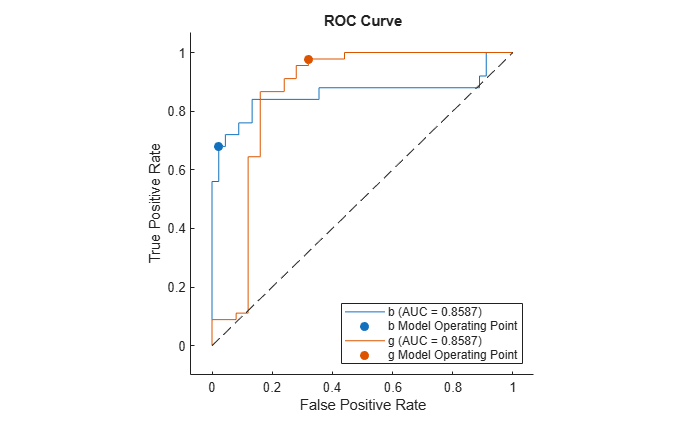For each class, the `plot` function plots a ROC curve and displays a filled circle marker at the model operating point. The legend displays the class name and AUC value for each curve.

Note that you do not need to examine ROC curves for both classes in a binary classification problem. The two ROC curves are symmetric, and the AUC values are identical. A TPR of one class is a true negative rate (TNR) of the other class, and TNR is 1-FPR. Therefore, a plot of TPR versus FPR for one class is the same as a plot of 1-FPR versus 1-TPR for the other class.

Plot the ROC curve for the first class only by specifying the `ClassNames` name-value argument.

`plot(rocObj,ClassNames=Mdl.ClassNames(1))`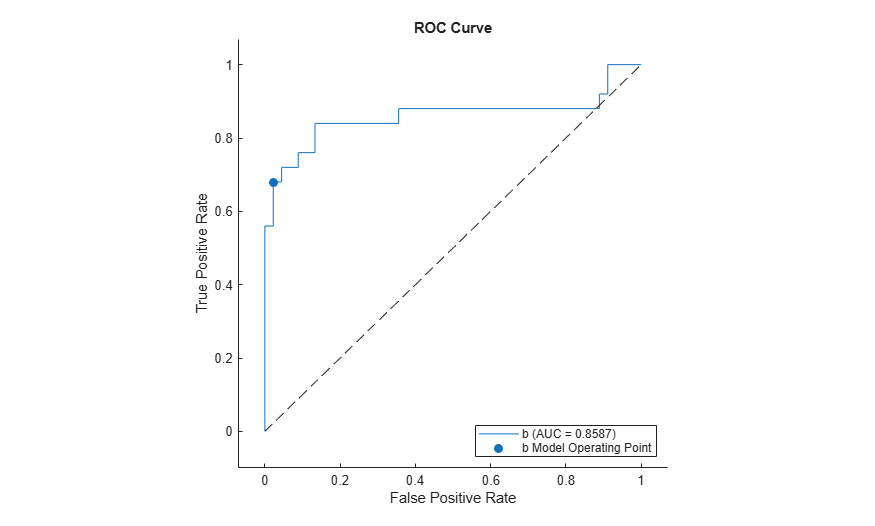Compute the performance metrics (FPR and TPR) for a multiclass classification problem by creating a `rocmetrics` object, and plot a ROC curve for each class by using the `plot` function. Specify the `AverageROCType` name-value argument of `plot` to create the average ROC curve for the multiclass problem.

Load the `fisheriris` data set. The matrix `meas` contains flower measurements for 150 different flowers. The vector `species` lists the species for each flower. `species` contains three distinct flower names.

`load fisheriris`

Train a classification tree that classifies observations into one of the three labels. Cross-validate the model using 10-fold cross-validation.

```rng("default") % For reproducibility Mdl = fitctree(meas,species,Crossval="on");```

Compute the classification scores for validation-fold observations.

```[~,Scores] = kfoldPredict(Mdl); size(Scores)```
```ans = 1×2 150 3 ```

The output `Scores` is a matrix of size `150`-by-`3`. The column order of `Scores` follows the class order in `Mdl`. Display the class order stored in `Mdl.ClassNames`.

`Mdl.ClassNames`
```ans = 3x1 cell {'setosa' } {'versicolor'} {'virginica' } ```

Create a `rocmetrics` object by using the true labels in `species` and the classification scores in `Scores`. Specify the column order of `Scores` using `Mdl.ClassNames`.

`rocObj = rocmetrics(species,Scores,Mdl.ClassNames);`

`rocObj` is a `rocmetrics` object that stores the AUC values and performance metrics for each class in the `AUC` and `Metrics` properties. Display the `AUC` property.

`rocObj.AUC`
```ans = 1×3 1.0000 0.9636 0.9636 ```

The table in `Metrics` contains the performance metric values for all three classes, vertically concatenated according to the class order. Find and display the rows for the second class in the table.

```idx = strcmp(rocObj.Metrics.ClassName,Mdl.ClassNames(2)); rocObj.Metrics(idx,:)```
```ans=13×4 table ClassName Threshold FalsePositiveRate TruePositiveRate ______________ _________ _________________ ________________ {'versicolor'} 1 0 0 {'versicolor'} 1 0.01 0.7 {'versicolor'} 0.95455 0.02 0.8 {'versicolor'} 0.91304 0.03 0.9 {'versicolor'} -0.2 0.04 0.9 {'versicolor'} -0.33333 0.06 0.9 {'versicolor'} -0.6 0.08 0.9 {'versicolor'} -0.86957 0.12 0.92 {'versicolor'} -0.91111 0.16 0.96 {'versicolor'} -0.95122 0.31 0.96 {'versicolor'} -0.95238 0.38 0.98 {'versicolor'} -0.95349 0.44 0.98 {'versicolor'} -1 1 1 ```

Plot the ROC curve for each class. Specify `AverageROCType="micro"` to compute the performance metrics for the average ROC curve using the micro-averaging method.

`plot(rocObj,AverageROCType="micro")`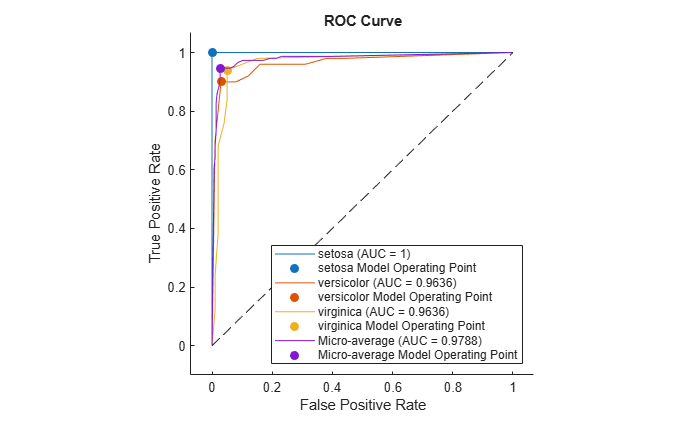The filled circle markers indicate the model operating points. The legend displays the class name and AUC value for each curve.

For generated samples containing outliers, train an isolation forest model and compute anomaly scores by using the `iforest` function. `iforest` returns scores as a vector. Use the scores to create a `rocmetrics` object. Plot the precision-recall curve using the anomaly scores, and find the model operating point for the isolation forest model.

Use a Gaussian copula to generate random data points from a bivariate distribution.

```rng("default") rho = [1,0.05;0.05,1]; n = 1000; u = copularnd("Gaussian",rho,n);```

Add noise to 5% of randomly selected observations to make the observations outliers.

```noise = randperm(n,0.05*n); true_tf = false(n,1); true_tf(noise) = true; u(true_tf,1) = u(true_tf,1)*5;```

Train an isolation forest model by using the `iforest` function. Specify the fraction of anomalies in the training observations as 0.05.

`[f,tf,scores] = iforest(u,ContaminationFraction=0.05);`

`f` is an `IsolationForest` object. `iforest` also returns the anomaly indicators (`tf`) and anomaly scores (`scores`) for the training data. `iforest` determines the threshold value (`f.ScoreThreshold`) so that the function detects the specified fraction of training observations as anomalies.

Check the performance of the `IsolationForest` object by plotting the precision-recall curve and computing the area under the curve (AUC) value. Create a `rocmetrics` object by using the true anomaly indicators (`true_tf`) and anomaly scores (`scores`). A score value close to 1 indicates an anomaly, as does the value `true` in `true_tf`. Therefore, specify the class name for `scores` as `true`. Specify the `AdditionalMetrics` name-value argument to compute the precision values (or positive predictive values).

`rocObj = rocmetrics(true_tf,scores,true,AdditionalMetrics="PositivePredictiveValue");`

Plot the curve by using the `plot` function of `rocmetrics`. Specify the y-axis metric as precision (or positive predictive value) and the x-axis metric as recall (or true positive rate). Display a filled circle at the model operating point corresponding to `f.ScoreThreshold`. Compute the area under the precision-recall curve using the trapezoidal method of the `trapz` function, and display the value in the legend.

```r = plot(rocObj,YAxisMetric="PositivePredictiveValue",XAxisMetric="TruePositiveRate"); hold on idx = find(rocObj.Metrics.Threshold>=f.ScoreThreshold,1,'last'); scatter(rocObj.Metrics.TruePositiveRate(idx), ... rocObj.Metrics.PositivePredictiveValue(idx), ... [],r.Color,"filled") xyData = rmmissing([r.XData r.YData]); auc = trapz(xyData(:,1),xyData(:,2)); legend(join([r.DisplayName " (AUC = " string(auc) ")"],""),"true Model Operating Point") xlabel("Recall") ylabel("Precision") title("Precision-Recall Curve") hold off```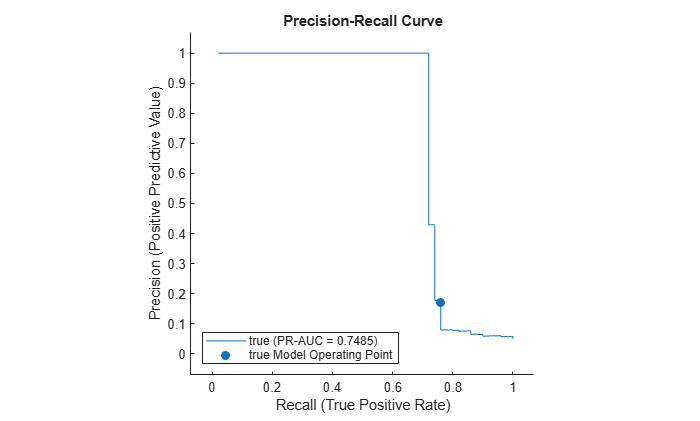Compute the confidence intervals for FPR and TPR for fixed threshold values by using bootstrap samples, and plot the confidence intervals for TPR on the ROC curve by using the `plot` function.

Load the `ionosphere` data set. This data set has 34 predictors (`X`) and 351 binary responses (`Y`) for radar returns, either bad (`'b'`) or good (`'g'`).

`load ionosphere`

Partition the data into training and test sets. Use approximately 80% of the observations to train a support vector machine (SVM) model, and 20% of the observations to test the performance of the trained model on new data. Partition the data using `cvpartition`.

```rng("default") % For reproducibility of the partition c = cvpartition(Y,Holdout=0.20); trainingIndices = training(c); % Indices for the training set testIndices = test(c); % Indices for the test set XTrain = X(trainingIndices,:); YTrain = Y(trainingIndices); XTest = X(testIndices,:); YTest = Y(testIndices);```

Train an SVM classification model.

`Mdl = fitcsvm(XTrain,YTrain);`

Compute the classification scores for the test set.

`[~,Scores] = predict(Mdl,XTest);`

Create a `rocmetrics` object by using the true labels in `YTest` and the classification scores in `Scores`. Specify the column order of `Scores` using `Mdl.ClassNames`. Specify `NumBootstraps` as 100 to use 100 bootstrap samples to compute the confidence intervals.

```rocObj = rocmetrics(YTest,Scores,Mdl.ClassNames, ... NumBootstraps=100);```

Find the rows for the second class in the table of the `Metrics` property, and display the first eight rows.

```idx = strcmp(rocObj.Metrics.ClassName,Mdl.ClassNames(2)); head(rocObj.Metrics(idx,:))```
``` ClassName Threshold FalsePositiveRate TruePositiveRate _________ _________ __________________________ ________________________________ {'g'} 7.1963 0 0 0 0 0 0 {'g'} 7.1963 0 0 0 0.022222 0 0.093023 {'g'} 6.2593 0 0 0 0.044444 0 0.11969 {'g'} 5.5728 0 0 0 0.066667 0.020988 0.16024 {'g'} 5.5642 0 0 0 0.088889 0.022635 0.18805 {'g'} 5.4619 0.04 0 0.22222 0.088889 0.022635 0.18805 {'g'} 5.3672 0.08 0 0.28 0.088889 0.022635 0.18805 {'g'} 5.1525 0.08 0 0.28 0.11111 0.045035 0.19532 ```

Each row of the table contains the metric value and its confidence intervals for FPR and TPR for a fixed threshold value. The `Threshold` variable is a column vector, and the `FalsePositiveRate` and `TruePositiveRate` variables are three-column matrices. The first column of the matrices corresponds to the metric values, and the second and third columns correspond to the lower and upper bounds, respectively.

Plot the ROC curve and the confidence intervals for TPR. Specify `ShowConfidenceIntervals=true` to show the confidence intervals, and specify one class to plot by using the `ClassNames` name-value argument.

`plot(rocObj,ShowConfidenceIntervals=true,ClassNames=Mdl.ClassNames(2))`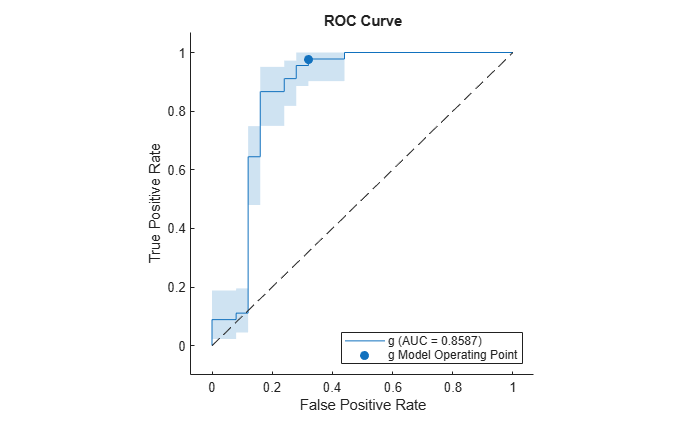The shaded area around the ROC curve indicates the confidence intervals. The confidence intervals represent the uncertainty of the curve due to the variance in the test set for the trained model.

Compute the confidence intervals for FPR and TPR for fixed threshold values by using cross-validated data, and plot the confidence intervals for TPR on the ROC curve by using the `plot` function.

Load the `fisheriris` data set. The matrix `meas` contains flower measurements for 150 different flowers. The vector `species` lists the species for each flower. `species` contains three distinct flower names.

`load fisheriris`

Train a naive Bayes model that classifies observations into one of the three labels. Cross-validate the model using 10-fold cross-validation.

```rng("default") % For reproducibility Mdl = fitcnb(meas,species,Crossval="on");```

Compute the classification scores for validation-fold observations.

`[~,Scores] = kfoldPredict(Mdl);`

Store the cross-validated scores and the corresponding true labels in cell arrays, so that each element in the cell arrays corresponds to one validation fold.

```cv = Mdl.Partition; numTestSets = cv.NumTestSets; cvLabels = cell(numTestSets,1); cvScores = cell(numTestSets,1); for i = 1:numTestSets testIdx = test(cv,i); cvLabels{i} = species(testIdx); cvScores{i} = Scores(testIdx,:); end```

Create a `rocmetrics` object using the cell arrays. If you specify true labels and scores by using cell arrays, `rocmetrics` computes the confidence intervals.

`rocObj = rocmetrics(cvLabels,cvScores,Mdl.ClassNames);`

Plot the ROC curve and the confidence intervals for TPR. Specify `ShowConfidenceIntervals=true` to show the confidence intervals.

`plot(rocObj,ShowConfidenceIntervals=true)`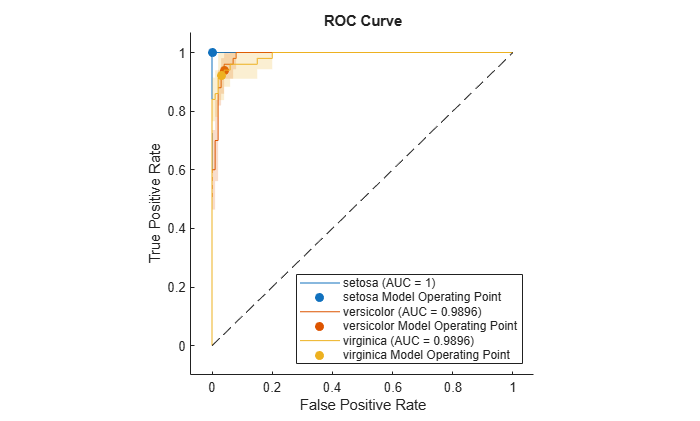The shaded area around each curve indicates the confidence intervals. The widths of the confidence intervals for `setosa` are 0 for nonzero false positive rates, so the plot does not have a shaded area for `setosa`. The confidence intervals reflect the uncertainty in the model due to the variance in the training and test sets.

Train three different classification models: decision tree model, generalized additive model, and naive Bayes model. Compare the performance of the three models on a test data set using the ROC curves and the AUC values.

Load the 1994 census data stored in `census1994``.mat`. The data set consists of demographic data from the US Census Bureau to predict whether an individual makes over \$50,000 per year.

`load census1994`

`census1994` contains the training data set `adultdata` and the test data set `adulttest`. Display the unique values in the response variable `salary`.

`classNames = unique(adultdata.salary)`
```classNames = 2x1 categorical <=50K >50K ```

Train the three models by passing the training data `adultdata` and specifying the response variable name `"salary"`. Specify the order of the classes by using the `ClassNames` name-value argument.

```MdlTree = fitctree(adultdata,"salary",ClassNames=classNames); MdlGAM = fitcgam(adultdata,"salary",ClassNames=classNames); MdlNB = fitcnb(adultdata,"salary",ClassNames=classNames);```

Compute the classification scores for the test data set `adulttest` using the trained models.

```[~,ScoresTree] = predict(MdlTree,adulttest); [~,ScoresGAM] = predict(MdlGAM,adulttest); [~,ScoresNB] = predict(MdlNB,adulttest);```

Create a `rocmetrics` object for each model.

```rocTree = rocmetrics(adulttest.salary,ScoresTree,classNames); rocGAM = rocmetrics(adulttest.salary,ScoresGAM,classNames); rocNB = rocmetrics(adulttest.salary,ScoresNB,classNames);```

Plot the ROC curve for each model. By default, the `plot` function displays the class names and the AUC values in the legend. To include the model names in the legend instead of the class names, modify the `DisplayName` property of the `ROCCurve` object returned by the `plot` function.

```figure c = cell(3,1); g = cell(3,1); [c{1},g{1}] = plot(rocTree,ClassNames=classNames(1)); hold on [c{2},g{2}] = plot(rocGAM,ClassNames=classNames(1)); [c{3},g{3}] = plot(rocNB,ClassNames=classNames(1)); modelNames = ["Decision Tree Model", ... "Generalized Additive Model","Naive Bayes Model"]; for i = 1 : 3 c{i}.DisplayName = replace(c{i}.DisplayName, ... string(classNames(1)),modelNames(i)); g{i}(1).DisplayName = join([modelNames(i),"Operating Point"]); end hold off```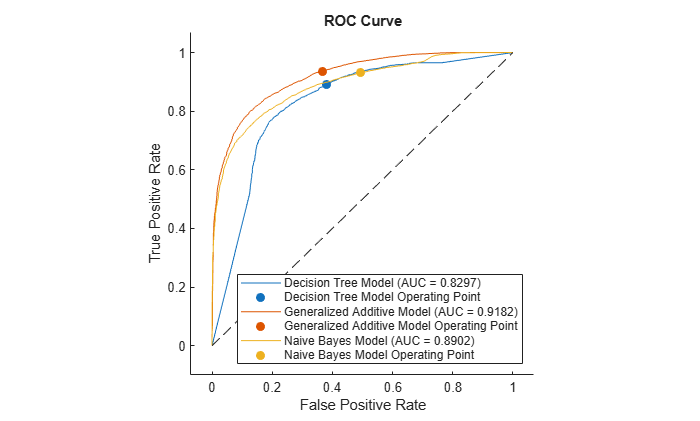The generalized additive model (`MdlGAM`) has the highest AUC value, and the decision tree model (`MdlTree`) has the lowest. This result suggests that `MdlGAM` has better average performance for the test data set than `MdlTree` and `MdlNB`.

Find the model operating point and the optimal operating point for a binary classification model. Classify observations in a test data set by using a new threshold corresponding to the optimal operating point.

Load the `ionosphere` data set. This data set has 34 predictors (`X`) and 351 binary responses (`Y`) for radar returns, either bad (`b`) or good (`g`).

`load ionosphere`

Partition the data into training and test sets. Use approximately 75% of the observations to train a support vector machine (SVM) model, and 25% of the observations to test the performance of the trained model on new data. Partition the data using `cvpartition`.

```rng("default") % For reproducibility of the partition c = cvpartition(Y,Holdout=0.25); trainingIndices = training(c); % Indices for the training set testIndices = test(c); % Indices for the test set XTrain = X(trainingIndices,:); YTrain = Y(trainingIndices); XTest = X(testIndices,:); YTest = Y(testIndices);```

Train an SVM classification model.

`Mdl = fitcsvm(XTrain,YTrain);`

Display the class order stored in `Mdl.ClassNames`.

`Mdl.ClassNames`
```ans = 2x1 cell {'b'} {'g'} ```

Compute the classification scores for the test set.

`[Y1,Scores] = predict(Mdl,XTest);`

Create a `rocmetrics` object by using the true labels in `YTest` and the classification scores in `Scores`. Specify the column order of `Scores` using `Mdl.ClassNames`.

`rocObj = rocmetrics(YTest,Scores,Mdl.ClassNames);`

Find the model operating point in the `Metrics` property of `rocObj` for class `b`. The `predict` function classifies an observation into the class yielding a larger score, which corresponds to the class with a nonnegative adjusted score. That is, the typical threshold value used by the `predict` function is 0. Among the rows in the `Metrics` property of `rocObj` for class `b`, find the point that has the smallest nonnegative threshold value. The point on the curve indicates identical performance to the performance of the threshold value 0.

```idx_b = strcmp(rocObj.Metrics.ClassName,"b"); X = rocObj.Metrics(idx_b,:).FalsePositiveRate; Y = rocObj.Metrics(idx_b,:).TruePositiveRate; T = rocObj.Metrics(idx_b,:).Threshold; idx_model = find(T>=0,1,"last"); modelpt = [T(idx_model) X(idx_model) Y(idx_model)]```
```modelpt = 1×3 1.2654 0.0179 0.5806 ```

For binary classification, an optimal operating point that minimizes the average misclassification cost is a point at which the ROC curve intersects a straight line with slope $m$, where $m$ is defined as

$\mathit{m}=\frac{\mathrm{cost}\left(\mathit{P}|\mathit{N}\right)-\mathrm{cost}\left(\mathit{N}|\mathit{N}\right)}{\mathrm{cost}\left(\mathit{N}|\mathit{P}\right)-\mathrm{cost}\left(\mathit{P}|\mathit{P}\right)}\cdot \frac{\mathit{n}}{\mathit{p}}$.

$p$ is the total number of observations in the positive class, and $n$ is the total number of observations in the negative class. The `cost` values are the components of the cost matrix $C$:

`$\mathit{C}=\left[\begin{array}{cc}\mathrm{cost}\left(\mathit{P}|\mathit{P}\right)& \mathrm{cost}\left(\mathit{N}|\mathit{P}\right)\\ \mathrm{cost}\left(\mathit{P}|\mathit{N}\right)& \mathrm{cost}\left(\mathit{N}|\mathit{N}\right)\end{array}\right]$`

`cost(N|P)` is the cost of misclassifying a positive class as a negative class, and `cost(P|N)` is the cost of misclassifying a negative class as a positive class. According to the class order in `Mdl.ClassNames`, the positive class `P` corresponds to class `b`.

Among the points on the ROC curve that intersect a line with slope $m$, choose one that is closest to the perfect classifier point (`FPR = 0`, `TPR = 1`), which the perfect ROC curve passes.

Find the optimal operating point for the positive class `b`.

```p = sum(strcmp(YTest,"b")); n = sum(~strcmp(YTest,"b")); cost = Mdl.Cost; m = (cost(2,1)-cost(2,2))/(cost(1,2)-cost(1,1))*n/p; [~,idx_opt] = min(X - Y/m); optpt = [T(idx_opt) X(idx_opt) Y(idx_opt)]```
```optpt = 1×3 -1.1978 0.1071 0.7742 ```

Plot the ROC curve for class `b` by using the `plot` function, and display the optimal operating point by using the `scatter` function.

```figure r = plot(rocObj,ClassNames="b"); hold on scatter(optpt(2),optpt(3),"filled", ... DisplayName="b Optimal Operating Point");```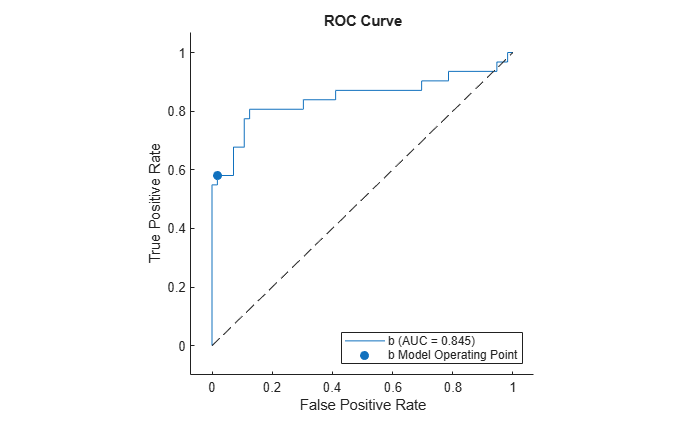Display the model operating point and the optimal operating point.

```array2table([modelpt;optpt], ... RowNames=["Model Operating Point" "Optimal Operating Point"], ... VariableNames=["Threshold" "FalsePositiveRate" "TruePositiveRate"])```
```ans=2×3 table Threshold FalsePositiveRate TruePositiveRate _________ _________________ ________________ Model Operating Point 1.2654 0.017857 0.58065 Optimal Operating Point -1.1978 0.10714 0.77419 ```

Classify `XTest` using the optimal operating point. Assign an observation whose adjusted score is greater than or equal to the optimal threshold to the positive class `b`.

```s = Scores(:,1) - Scores(:,2); idx_b_opt = (s >= optpt(1)); Y2 = cell(size(YTest)); Y2(idx_b_opt) = {'b'}; Y2(~idx_b_opt) = {'g'};```

Display the adjusted scores for the observations that have different labels in `Y1` (labels from the `predict` function) and `Y2` (labels from the optimal threshold `optpt(1)`).

`s(~strcmp(Y1,Y2))`
```ans = 11×1 -1.1703 -0.8445 -0.8235 -0.4546 -1.0719 -0.4612 -0.2191 -1.1978 -1.0114 -1.1552 ⋮ ```

Eleven observations have adjusted scores less than `0` but greater than or equal to the optimal threshold.

After training a model for a multiclass classification problem, create a `rocmetrics` object for classes of interest only. Specify `FixedMetricValues` so that `rocmetrics` computes the performance metrics for the specified threshold values.

Read the sample file `CreditRating_Historical.dat` into a table. The predictor data consists of financial ratios and industry sector information for a list of corporate customers. The response variable consists of credit ratings assigned by a rating agency. Preview the first few rows of the data set.

```creditrating = readtable("CreditRating_Historical.dat"); head(creditrating)```
``` ID WC_TA RE_TA EBIT_TA MVE_BVTD S_TA Industry Rating _____ ______ ______ _______ ________ _____ ________ _______ 62394 0.013 0.104 0.036 0.447 0.142 3 {'BB' } 48608 0.232 0.335 0.062 1.969 0.281 8 {'A' } 42444 0.311 0.367 0.074 1.935 0.366 1 {'A' } 48631 0.194 0.263 0.062 1.017 0.228 4 {'BBB'} 43768 0.121 0.413 0.057 3.647 0.466 12 {'AAA'} 39255 -0.117 -0.799 0.01 0.179 0.082 4 {'CCC'} 62236 0.087 0.158 0.049 0.816 0.324 2 {'BBB'} 39354 0.005 0.181 0.034 2.597 0.388 7 {'AA' } ```

Because each value in the `ID` variable is a unique customer ID, that is, `length(unique(creditrating.ID))` is equal to the number of observations in `creditrating`, the `ID` variable is a poor predictor. Remove the `ID` variable from the table, and convert the `Industry` variable to a `categorical` variable.

```creditrating = removevars(creditrating,"ID"); creditrating.Industry = categorical(creditrating.Industry);```

Partition the data into training and test sets. Use approximately 80% of the observations to train a neural network model, and 20% of the observations to test the performance of the trained model on new data. Partition the data using `cvpartition`.

```rng("default") % For reproducibility of the partition c = cvpartition(creditrating.Rating,"Holdout",0.20); trainingIndices = training(c); % Indices for the training set testIndices = test(c); % Indices for the test set creditTrain = creditrating(trainingIndices,:); creditTest = creditrating(testIndices,:);```

Train a neural network classifier by passing the training data `creditTrain` to the `fitcnet` function.

`Mdl = fitcnet(creditTrain,"Rating");`

Compute classification scores and predict credit ratings for the test set observations.

`[labels,Scores] = predict(Mdl,creditTest);`

The classification scores for a neural network classifier correspond to posterior probabilities.

Assume that you want to evaluate the model only for the ratings `B`, `BB`, and `BBB`, and ignore the rest of the ratings.

Display the order of the ratings in the model stored in the `ClassNames` property, and identify the classes to evaluate.

`Mdl.ClassNames`
```ans = 7x1 cell {'A' } {'AA' } {'AAA'} {'B' } {'BB' } {'BBB'} {'CCC'} ```
```idx_Class = [4 5 6]; classesToEvaluate = Mdl.ClassNames(idx_Class);```

Find the indices of the observations for the three classes (`B`, `BB`, `BBB`).

`idx = ismember(creditTest.Rating,classesToEvaluate);`

Create a `rocmetrics` object using the true labels and scores for the three classes. Specify `FixedMetricValues=1:-0.25:-1` so that `rocmetrics` computes the performance metrics for the specified threshold values.

```thresholds = 1:-0.25:-1; rocObj = rocmetrics(creditTest.Rating(idx),Scores(idx,idx_Class), ... classesToEvaluate,FixedMetricValues=thresholds);```

Display the computed metrics stored in the `Metrics` property.

`rocObj.Metrics`
```ans=27×4 table ClassName Threshold FalsePositiveRate TruePositiveRate _________ _________ _________________ ________________ {'B' } 0.8987 0 0 {'B' } 0.81356 0 0.09375 {'B' } 0.50257 0.007732 0.3125 {'B' } 0.25635 0.025773 0.42188 {'B' } 0.014391 0.051546 0.57812 {'B' } -0.22962 0.11082 0.6875 {'B' } -0.48407 0.17526 0.75 {'B' } -0.74996 0.51804 0.84375 {'B' } -0.97442 1 1 {'BB'} 0.96327 0 0 {'BB'} 0.75156 0.052434 0.19459 {'BB'} 0.50483 0.10861 0.44324 {'BB'} 0.25408 0.14981 0.60541 {'BB'} 0.0055626 0.22846 0.74595 {'BB'} -0.23851 0.33708 0.84324 {'BB'} -0.49618 0.47191 0.94595 ⋮ ```

The `Metrics` property contains the performance metrics for the three ratings `B`, `BB`, and `BBB` and the specified threshold values only. The default `UseNearestNeighbor` value is `true` if `rocmetrics` does not compute confidence intervals. Therefore, for each specified threshold value, `rocmetrics` selects an adjusted score value nearest to the specified value and uses the nearest value as a threshold. Display the specified threshold values and the actual threshold values used for each class.

```idx_B = strcmp(rocObj.Metrics.ClassName,"B"); idx_BB = strcmp(rocObj.Metrics.ClassName,"BB"); idx_BBB = strcmp(rocObj.Metrics.ClassName,"BBB"); table(thresholds',rocObj.Metrics.Threshold(idx_B), ... rocObj.Metrics.Threshold(idx_BB), ... rocObj.Metrics.Threshold(idx_BBB), ... VariableNames=["Fixed Threshold";string(classesToEvaluate)])```
```ans=9×4 table Fixed Threshold B BB BBB _______________ ________ _________ _________ 1 0.8987 0.96327 0.93863 0.75 0.81356 0.75156 0.7516 0.5 0.50257 0.50483 0.50177 0.25 0.25635 0.25408 0.25086 0 0.014391 0.0055626 0.0039881 -0.25 -0.22962 -0.23851 -0.24539 -0.5 -0.48407 -0.49618 -0.49944 -0.75 -0.74996 -0.74338 -0.74854 -1 -0.97442 -0.93863 -0.96909 ```

expand all

## Algorithms

expand all

 Fawcett, T. “ROC Graphs: Notes and Practical Considerations for Researchers”, Machine Learning 31, no. 1 (2004): 1–38.

 Zweig, M., and G. Campbell. “Receiver-Operating Characteristic (ROC) Plots: A Fundamental Evaluation Tool in Clinical Medicine.” Clinical Chemistry 39, no. 4 (1993): 561–577.

 Davis, J., and M. Goadrich. “The Relationship Between Precision-Recall and ROC Curves.” Proceedings of ICML ’06, 2006, pp. 233–240.

 Moskowitz, C. S., and M. S. Pepe. “Quantifying and Comparing the Predictive Accuracy of Continuous Prognostic Factors for Binary Outcomes.” Biostatistics 5, no. 1 (2004): 113–27.

 Huang, Y., M. S. Pepe, and Z. Feng. “Evaluating the Predictiveness of a Continuous Marker.” U. Washington Biostatistics Paper Series, 2006, 250–61.

 Briggs, W. M., and R. Zaretzki. “The Skill Plot: A Graphical Technique for Evaluating Continuous Diagnostic Tests.” Biometrics 64, no. 1 (2008): 250–256.

 Bettinger, R. “Cost-Sensitive Classifier Selection Using the ROC Convex Hull Method.” SAS Institute, 2003.

 DiCiccio, Thomas J., and Bradley Efron. “Bootstrap Confidence Intervals.” Statistical Science 11, no. 3 (1996): 189–228.

 Efron, Bradley, and Robert J. Tibshirani. An Introduction to the Bootstrap. New York: Chapman & Hall, 1993.

 Efron, Bradley. The Jackknife, the Bootstrap and Other Resampling Plans. Philadelphia: The Society for Industrial and Applied Mathematics, 1982.

 Davison, A. C., and D. V. Hinkley. Bootstrap Methods and Their Applications. Cambridge University Press, 1997.